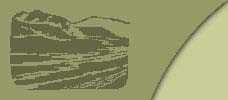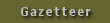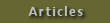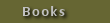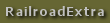CHAPTER XX. By -. G. A. Aleyer. LAYING OUT LINK-MOTION. FIG. 19 is an outline of a link-motion such as is generally applied to the American locomotive. It can be adjusted to control the movement of the slide-valve in such a manner that equal portions of steam will be admitted alternately at each end of the cylinder.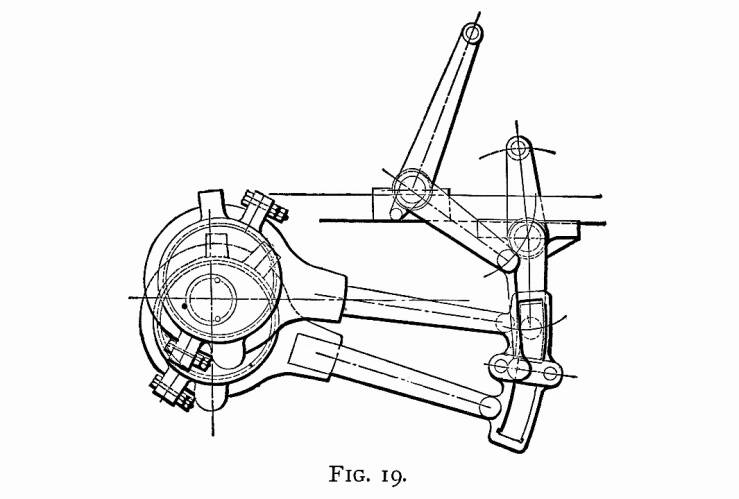In the following article we propose to explain how this can be accomplished. Although we would not advise any person to be satisfied with approximate rules or constructions, yet cases do occur where the approximate constructions, being so very near correct, on account of their simplicity, are of greater practical value than the application of the rigid and more difficult theoretical rules. By these remarks, we do not wish the reader to understand that the following constructions are all done according to the rules of thumb — not by any means; for all, with the exception of a few points, are theoretically correct. At the end of this article, we will point out those points which are, and which are not, approximately found; so that the reader may feel satisfied that our construction may always be relied upon as being correct for all practical purposes. In what follows, the cylinder will always be regarded as lying on the right-hand side of the axle, the link being between cylinder and axle, and the axle located in the center of pedestal. To avoid any misunderstanding, we will explain the meaning of some of the terms used. The length of crank is the distance from center of axle to center of crank-pin. For convenience, we shall call the total distance from center of eccentric-strap to the center of eccentric-pin in the link the length of the eccentric-rod. The throw of eccentric is double the distance from center of axle to center of eccentric-wheel. The length of the connecting rod is the distance from the center of crank-pin hole to center of cross-head pin-hole. The length of link-hanger is the distance from center to center of holes. CONDITIONS. Since this article treats only on the adjustment of the link-motion, the following items are supposed to be known and established: The lap of valve, which in this case will be three-fourths of an inch; the throw of eccentrics, 5 inches; the stroke of the piston, 24 inches; the position of the rocker, as per Fig. 19; the length of the rocker-arms, which are in this case of equal length; length of link-hanger and all dimensions of link, complete, as shown in Fig. 19 and also the length of the connecting rod. The adjustment of the link-motion may at first sight appear to be a difficult problem, as we must have a knowledge of the relative motions of the piston and slide-valve; but by reducing this problem to several elementary problems, so that the laws governing the relative motions may be discovered and clearly defined, a clear conception of our subject can be gained, and the solution of our original problem can be accomplished with comparative ease. In order to find what kind of elementary problems are applicable, let us suppose that we are looking at a locomotive with a link-motion, as shown in Fig. 19, applied and correctly adjusted. Now let us examine it, commencing with the valve. We find that the valve receives its motion from the upper rocker-arm, and this receives its motion from the lower rocker-arm. According to our conditions, previously stated, both of the rocker-arms are of the same length; and, therefore, the arc described by the upper rocker-arm will be the same length as the arc described by the lower one. We also notice that the link which moves the lower rocker-arm is held in position by the lifting-shaft arm. The question, then, will naturally arise, Must this lifting-shaft arm have some particular length, and the center of lifting-shaft have some particular position? We answer, "Yes;" and this is one of our elementary problems to solve. Again, we notice that the saddle-pin is not in the center of the link; and we ask again, "Why?" To answer this will be another elementary problem. The next we notice are our eccentric-rods. These we find, on examination, to have some particular length; and to find this length is another elementary problem. Next we examine our eccentrics: these, we find, are fastened to the axle; and, since the crank is also fastened to the same axle, it follows that there are some relative positions between them; to find these positions is another elementary problem. Now let us look once more at the rocker, and we find that the two rocker-arms are not in the same straight line: hence, to find the amount of offset is another elementary problem. And, lastly, we must be able to find the position of crank-pin to correspond with the position of piston when at full stroke at either end of the cylinder, and also when at half stroke moving in either direction. Here, then, we have all the elementary problems that are necessary to be understood for the solution of our original problem. We will now explain all these problems, in an order the reverse to that in which we stated them: hence we have the following order — 1st, To find position of crank at full and half stroke. 2d, To find center line of motion, and amount of offset in rocker-arms. 3d, To find relative positions of crank-pin and eccentrics when at full and half stroke. 4th, To determine the correct length of eccentric-rods. 5th, To find position of saddle-pin. 6th, To find the position of the center of lifting-shaft and length of arms. PROBLEM 1, FIGS. 20 and 21.—To find the position of crank when the piston is at full and half stroke.— Let the center of wheel and the axis of the cylinder be in the same straight line as AD, Fig. 20. With any point C as a center, and a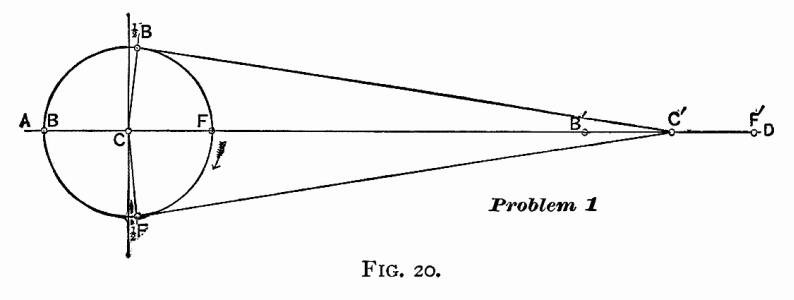radius equal to the length of the crank, describe a circle F, 2F, B, 2B; and let us call this the crank-pin circle. The straight line AD intersects the circumference of the circle in the points F and B. The point F will be the center of crank-pin when piston is at full stroke at the forward end of the cylinder, and point B will be the center of crank-pin when the piston is at full stroke at the rear end of the cylinder. With the point F as a center, and with a radius equal to the length of the connecting rod, describe an arc intersecting the line AD in the point F'; with the point B as a center, and with the same radius, describe an arc intersecting the straight line AD in the point B'; and with the point C as a center, and with the same radius, describe an arc intersecting the straight line AD in the point C. Point F' will be the center of cross-head pin when the center of crank-pin is at F, and B' the center of cross-head pin when the crank-pin is at B, and the point C' will be the position of center of cross-head pin when piston is at half stroke. With point C' as a center, and a radius equal to the length of the connecting rod, describe an arc passing through the point C, and intersecting the crank-pin circle in the points 2F and 2B: these points will be the position of crank-pin when the piston is at half stroke, or when the center of cross-head pin is at C'.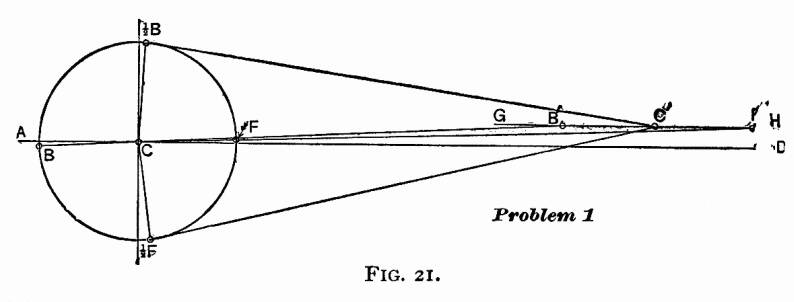It often happens that the axis of the cylinder is above the center of axle. When such is the case, we must follow the construction as shown in Fig. 21. Let two inches be the distance that the center of axis of cylinder is above the center of axle. Draw a straight line AD through the center of axle C; two inches above this draw a straight line GH parallel to AD; this line will then pass through the axis of cylinder. With the center of axle C on the straight line AD as a center, and a radius equal to the length of the crank, describe a circle F, 2F, B, 2B: this circle will be the crank-pin circle. With the point C as a center, and a radius equal to the length of the connecting rod plus the length of the crank, describe an arc intersecting the straight line GH in the point F': this point will be the position of the cross-head pin when the piston is at full stroke forward. Through the points F' and C draw a straight line, intersecting the crank-pin circle in the point F: this point will be the position of the center of the crank-pin when the piston is at full stroke forward. Again, with the point C as a center, and a radius equal to the length of, the connecting rod minus the length of the crank, describe an arc intersecting the straight line GH in the point B': this point will be the position of the center of the cross-head pin when the piston is at full stroke in the rear end of the cylinder. Through the points B and C draw a straight line, intersecting the crank-pin circle in the point B: this point will be the position of the center of crank-pin when the piston is at full stroke in the rear end of the cylinder. Find a point C' exactly central between the points B' and F' on the line GH: in other words, bisect the distance B' F' by the point C'. With the point C' as a center, and a radius equal to the length of the connecting rod, describe an arc intersecting the crank-pin circle in the points 2B and ½F: these two points will be the center of crank-pin when the piston stands at half stroke. In the link-motion, as shown in Fig. 19 the axis of the cylinder is supposed to be 2 inches higher than the center of axle. For this reason the construction shown in Fig. 21 will hereafter be used. PROBLEM 2, FIG. 22. — To find the center line of motion and the amount of offset in the lower rocker-arm. — Let C be the centre of axle: through C draw the straight lines AD and KL perpendicular to AD. The center of rocker we find in Fig. 19 to be 372 inches in front of the center of axle, and 72 inches above. We therefore continue our construction in Fig. 22 by drawing a straight line MN 372 inches in front of, and parallel to, the straight line KL, and another straight line OP parallel to AD, and 72 inches above it. These two lines intersect in the point Q, and this point is the center of rocker. With Q as a center, and a radius equal to the length of the lower rocker-arm, describe the arc RS: through the point C draw a straight line CT tangent to the arc RS, then CT will be the center line of motion.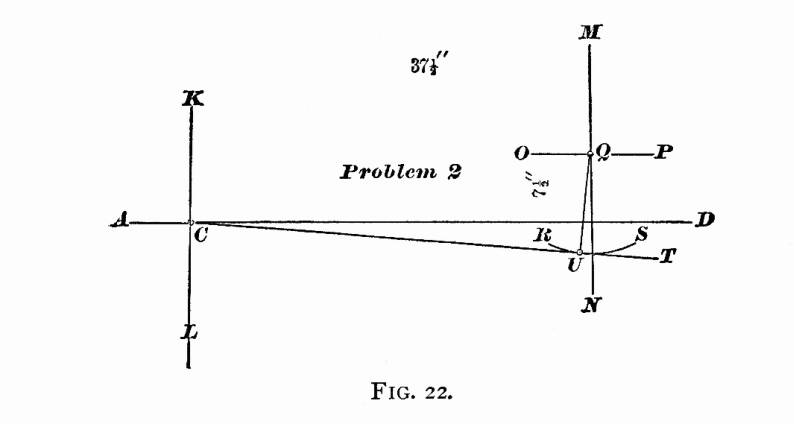To find the amount of offset in the lower rocker-arm, let us place the center line of the upper rocker-arm perpendicular to a line drawn parallel to the valve surface: but in our case this valve surface is parallel to the line AD; hence our line drawn parallel to the valve surface will also be parallel to the line AD, and the center line of upper rocker-arm will be perpendicular to AD, and coincide with the line MN. Through the point Q draw a straight line perpendicular to the line CT, and intersecting the arc RS in the point U: then the distance from the point U to the line MN will be the amount of the offset in the lower rocker-arm. PROBLEM 3, FIG. 23. — To find the relative positions of crank-pin and eccentrics when the piston is at full and half stroke. — Let C be the center of axle. Through C draw the horizontal line AD, and find the positions of center of crank-pin at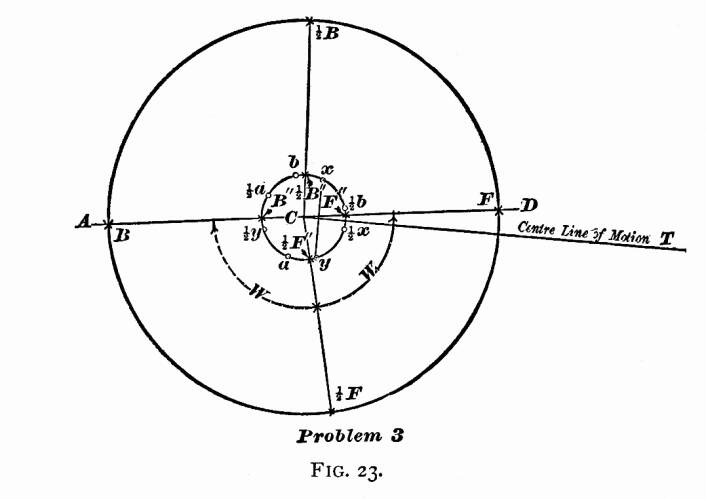full and half stroke; namely, the points F, 2F, 2B, B as explained in Problem 1 and shown in Fig. 21. Next draw the center line of motion as explained in Problem 2 and Fig. 22. With the point C as a center, and a radius equal to 2 the throw of the eccentric (22 inches), draw a circle; and let us call this circle the "eccentric-circle." On the line of motion CT, lay off a point towards the rocker M of an inch from C (this being the sum of the lap and lead,— w of an inch for the lap, and z of an inch for the lead) through this point draw a straight line perpendicular to the line of motion CT, and intersecting the eccentric-circle in the points x and y. The point x will be the center of the forward eccentric; and the point y will be the center of backward eccentric when the center of crank-pin is at F, full stroke forward. Through the points F and C draw a straight line, intersecting the eccentric-circle in the point F". The line FC will represent the center line of crank; and the distance between the points F" and x, measured on the eccentric-circle, is the amount that the center of forward eccentric is set back of the center line of crank; and the distance between the points F" and y is the amount that the backward eccentric is set ahead of the center line of crank. Since both the crank and eccentrics are fastened to the same axle, it follows, that, whatever position the center line of crank may be in, the distances between center line of crank and eccentrics — that is, the distances between F'' and x, also F" and y, measured on the eccentric-circle—remain constant. Therefore, to find the position of eccentrics when the crank stands at 2F (half stroke), draw the straight line 2 FC representing the center line of crank, and intersecting the eccentric-circle in the point 2F". From the point 2F", lay off on the eccentric-circle a point with a distance equal to F" x, back of the center line of crank, and indicate this point by 2x; also from 2F" measured on the same circle, lay off a point in the front of the center line of crank, and with a distance equal to F" y and mark this point 2y; then the point 2x will be the position of forward eccentric, and the point 2y will be the position of backward eccentric when the crank-pin is at 2F. In precisely the same manner we find the position of eccentrics when the center of crank-pin is at B (full stroke back). Through the points C and B draw a straight line, intersecting the eccentric-circle in the point B". From the point B", and with a distance equal to F" x lay off a point on the eccentric-circle back of crank; this point will be the position of forward eccentric when crank is at full stroke back; and, in order to distinguish this from the other position of eccentric, call this point a: also from B", lay off in front of the crank the position of backward eccentric at a distance equal to F" y and call this point b. In the same manner find points 2a and 2b when the crank-pin is at 2B. We have now found the position of eccentrics when the crank-pin stands in the following positions:— Full stroke forward F, the forward eccentric will be at x. Half stroke forward ½F, the forward eccentric will be at 2x. Full stroke back end B, the forward eccentric will be at a. Half stroke back end 2B, the forward eccentric will be at 2a. Full stroke forward F, the backward eccentric will be at y. Half stroke forward ½F, the backward eccentric will be at 2y. Full stroke back end B, the backward eccentric will be at b. Half stroke back end 2B the backward eccentric will be at 2b.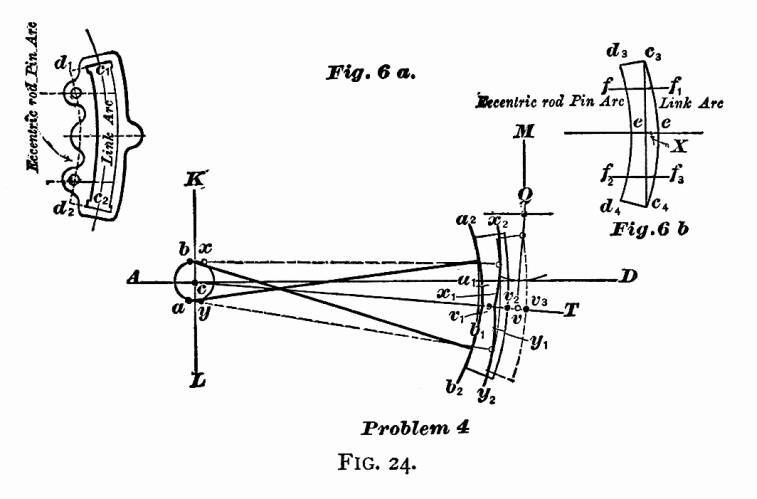PROBLEM 4, FIG. 24. — To determine the correct length of the eccentric-rods. — Let c be the center of axle. Through this point draw the horizontal line AD, also a line KL perpendicular to AD. The only purpose for which these two lines are drawn in this problem, as well as the others, is to have some lines from which we can locate other lines or points. Locate the center of rocker, and center lines of rocker-arms, as explained in Problem 2, and shown in Fig. 22; the lower arm standing perpendicular to the center line of motion, and the upper arm vertical. When the arms stand in this position, the rocker-pins will move through an equal distance on each side of these center lines during the time that the valve is making its full travel. Next find centers of eccentrics x and y when the crank is at full stroke forward; also a and b when the crank is at full stroke back, as explained in Problem 3, and shown in Fig. 23. Before we proceed, let us give names to some of the lines, as shown in Fig. 6a. The arc c1 c2 drawn through the center of opening of the link, we will call the link-arc; and the arc d1 d2 drawn through the center of eccentric-rod pin-holes, we will call the eccentric-rod pin-arc. Both of these arcs are drawn from the same center; that is, the center from which the link is drawn. Let us now cut a paper template, as shown in Fig. 6b (link structure). This template is cut so that, if it is laid on the link, Fig. 6a, the arc of the template c3 c4 will coincide with the link-arc c1 c2 and d3 d4 with the eccentric-pin arc d1 d2 the end of template d3 c3 with the line d1 c1, and the end d4 c4 with d2 c2. On this template join the points c3 c4 by a straight line, and bisect this line by the perpendicular line ee: on this line the center of the saddle-pin will be located. On one side of this line draw the line ff1 parallel to ee, and on the other side draw f2 f3 also parallel to ee; the distance from the point f to the point f2 being equal to the distance between the centers of eccentric-rod pins, and f e equal to e f2. The points f and f2 on the arc d3 d4 indicate the position on the template of the centers of eccentric-rod pins. On the center line of motion c T, lay off from v a point v1 towards the axle, with a distance equal to c1 d1, Fig. 6a; then with the point x as a center, and cv1 as a radius, describe the arc x1 x2; in this arc the upper eccentric-rod will be located as long as the center of forward eccentric remains at x. With the point y as a center, and cv1 as a radius, describe the arc y1 y2: in this arc the center of lower eccentric-rod will be located as long as the backward eccentric remains at y. With the point a as a center, and cv1 as a radius, describe an arc a1 a2: in this arc the upper eccentric-rod pin will be located while the forward eccentric is at a. With the point b as a center, and cv1 as a radius, describe the arc b1 b2; and in this arc the center of lower eccentric-rod pin will be located when the backward eccentric is at b. Now adjust the template on the drawing so that the point f will be in the arc x1 x2: point f2 in the arc y1 y2 and the line ee coincide with the center line of motion c T. Along the arc c3 c4 of the template draw an arc on the paper. Next move the template so that the point f will be in the arc a1 a2, the point f2 in the arc b1 b2 and the line ee coincide with the center line of motion c T, and along the arc c3 c4 of the template draw the second arc on the paper. Now, if the distance measured on the arc RS from the point v (the center of the lower rocker-arm pin) to the first arc drawn, is equal to the distance measured on the arc RS from v to the second arc, the radius cv1 will be the correct length of the eccentric-rods. But, if the distance from v to the first arc is less than the distance from v to the second arc, the length cv1 of the eccentric-rod will be too short. In this case we must increase the length cv1 by adding an amount equal to one-half the difference of the distances from v to the first arc, and from v to the second arc previously drawn; and this last length so found will be the correct length of eccentric-rods. It will be proper to remark here, that the radius cv1 was assumed to be the correct length of eccentric-rods; but since the rods cross each other when the eccentrics are at a and b, and do not cross each other when at x and y, the radius cv1 will always be a trifle short. It is therefore necessary to make the correction as explained. In every case, the length of eccentric-rods must be so adjusted, that, when the line ee coincides with the center line of motion cT, the arc vv2 (which is the amount that the rocker-pin is drawn towards the axle from the line Qv when the eccentrics are at a and b) must be equal to the arc vv3 (which is the amount that the rocker-pin is moved towards the cylinders from the line Qv when the eccentrics are at x and y); the straight line Qv being perpendicular to the center line of motion cT. PROBLEM 5, FIG. 25. — To find the position of the center of saddle-pin. — For this problem we again call to our aid the paper template shown in Fig. 6b. We have already seen in Problem 4 that the center of saddle-pin will be located on the line ee drawn on this template: it now only remains to determine the distance of this point from the link-arc c3 c4. Since the inequality between the crank-angle W and W1, Fig. 23, becomes the greatest when the crank stands at half stroke, it is of the utmost importance to find such a position for the center of saddle-pin that equal portions of steam will be admitted alternately when the crank stands at half stroke. Or, in other words, the admittance of steam must cease at the moment that the piston has completed one-half stroke.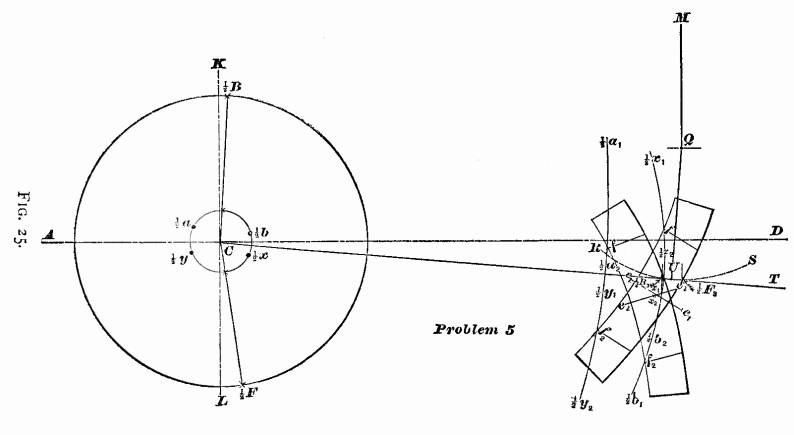Let us commence this problem as we began the others; namely, Through the center of axle C, Fig. 25, draw the horizontal line AD, also the vertical line LK. Find the position of crank at half stroke, as shown in Fig. 21. Next find the position of center line of motion CT, and position of rocker, as shown in Fig. 22. Find the relative position of eccentrics and crank when at half stroke, as shown in Fig. 23. Now, with a radius equal to the correct length of eccentric-rods, previously determined (shown in Fig. 24), describe from the point 2x as a center the arc 2x1 2x2; also with the point 2y as a center, and with the same radius, the arc 2y1 2y2. Again, from the point 2a as a center, describe the arc 2a1 2a2; also with the point 2b as a center, describe the arc 2b1 2b2 using the length of eccentric-rods as a radius for all the arcs. When the center of the forward eccentric is at 2x, the forward eccentric-rod pin will be located in the arc 2x1 2x2. When the center of the forward eccentric is at 2a, the forward. eccentric-rod pin will be located in the arc 2a1 2a2. When the backward eccentric is at 2y, its eccentric-rod pin will be located in the arc 2y1 2y2. When the center of the backward eccentric is at 2b, the eccentric-rod pin will be located in the arc 2b1 2b2. The next step is to find the relative position of the lower rocker-arm pin when steam is cut off at half stroke.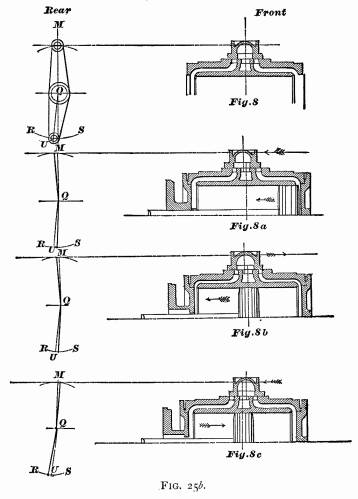In Fig. 25b 8 we have placed the slide-valve centrally over the ports, that is, it laps over each steam-port an equal amount, namely, w of an inch, which is equal to the lap. In this position of the valve, the center line of the upper rocker-arm will stand perpendicular to the line drawn parallel to the valve-face, and the center line of the lower rocker-arm will stand perpendicular to the center line of motion CT: hence the center line of upper rocker-arm MQ in Fig. 25b 8 will coincide with the line MQ in Fig. 25, and the center of lower arm QU in Fig. 25b 8 will coincide with the line QU in Fig. 25. Now let us follow the relative movement of the valve and piston. We find, that, when the piston commences its backward motion, the valve moves in the same direction, as shown by the arrow-points in Fig. 25b 8a; and, during the time that the piston is completing the half stroke, the valve has finished its full travel backward, and commenced moving forward, as indicated by the arrow-points, Fig. 25b 8b; and, at the time that the piston stands exactly at half stroke, the forward edge of the valve is just closing the forward steam-port, and consequently cutting off steam at half stroke when the piston is moving backward. From this we see, that, when the piston has completed the half stroke when moving backward, the center of the valve will be a little in the rear of the center of exhaust-port; the distance between the center of valve and the center of exhaust-port being w of an inch, the amount of the lap: the upper rocker-pin will stand w of an inch behind the line MQ and the lower rocker-arm pin will be w of an inch in front of the line QU, as shown in Fig. 25b 8b. We therefore draw in Fig. 25 a straight line parallel to QU, and w of an inch in front of it: this line will intersect the arc RS in the point 2F3; and this point is the position of the center of lower rocker-arm pin when the crank stands at 2F, and steam cut off at half stroke. Let the piston complete its backward stroke, and then commence moving forward towards half stroke, as shown by the arrow-point, Fig. 25b 8c. During this time the valve has completed its full travel forward, and commenced traveling backward, as indicated by the arrow-point, Fig. 25b 8c; and, when the piston stands exactly at half stroke, the rear edge of the valve is just closing the rear steam-port, and consequently cutting off steam at half stroke when the piston is moving forward. In this position the center line of the valve will be w of an inch in front of the center of exhaust, the center of the upper rocker-arm pin will be w of an inch in front of the line MQ, and lower rocker-pin w of an inch in the rear of the line QU, as shown in Fig. 25b 8c. We therefore draw in Fig. 25 a line parallel to QU, and w of an inch behind it; this line will intersect the arc RS in the point 2B3; and this point will be the position of the center of lower rocker-arm pin when the crank stands at 2B, and steam cut off at half stroke. Now, remember, that when the crank stands at 2F, Fig. 25, the forward eccentric will be at 2x, and the backward eccentric at 2y; and, if the link is raised or lowered while the eccentrics remain at 2x 2y, the forward eccentric-rod pin will move in the arc 2x1 2x2 and the backward eccentric-rod pin will move in the arc 2y1 2y2. Let us now find the position of link when steam is cut off at half stroke at either end of the cylinder. The points 2F3 and 2B3 in Fig. 25 being located, place the paper template on the drawing so that the point f will lie in the arc 2x1 2x2, and the point f2 in the arc 2y1 2y2, and the link-arc c3c4 just touching the point 2F3. While the template is in this position, draw on the paper along the edge c3c4 a portion of the link-arc, and mark the position that the line ee occupied, so that, when the template is removed, the line e1e1 can be drawn on the paper to represent the line ee of the template. Next place the template so that the point f will lie in the arc ½a1 ½a2 the point f2 in the arc ½b1 ½b2 and the link-arc c3c4 just touching the point ½B3 and, while in this position, draw part of the link-arc c3c4 on the paper, mark the position that the line ee occupied, and, after the template is removed, draw the line e2e2 on the paper to represent the line ee of the template. Now find by trial a point x1 on the line e1e1, and another point x2 on the line e2e2, so that the distances of these points from their link-arcs are equal, and that a straight line drawn through them will be parallel to the center line of motion. The distance from x1 to the link-arc—or, which is the same thing, the distance from the point x2 to the link-arc—will be the correct distance between the center of saddle-pin and the link-arc c1c2, Fig. 6a. Or, in other words, the position of the point x1, or x2, Fig. 25, will indicate the proper position of the point of suspension on the link. For future reference, let us mark this point of suspension on the template, and indicate it by X, Fig. 6b. PROBLEM 6, FIG. 26. — To find the position of the center of lifting-shaft and the length of its arms.—In the last problem we found the point of suspension of the link, so that it will cause the valve to cut off equal portions of steam when the piston stands at half stroke. It now remains for us to find the position of the lifting-shaft and the length of the lifting-shaft arms, so that the greatest equal amounts of steam will be admitted alternately at each end of the cylinder. Here a little difficulty arises which needs explanation, so that our construction may not seem inconsistent to the reader. It would be an easy matter to place our lifting-shaft to accomplish the object just stated; but, if we do this, the lead will not be equal at each end of cylinder when the piston is at full stroke. Again, if we locate our lifting-shaft in such a manner that equal lead will be obtained, then the maximum cut-off will not be equal; but the difference will be comparatively so small that it will not injure the working of the engine. This small difference of the maximum cut-off is therefore considered among practical men of little or no importance, but it is always considered good practice to have an equal lead at full stroke. Let us therefore adjust the lifting-shaft to obtain an equal lead, and allow us to consider the maximum cut-off to be equal when the lead is equal at full stroke.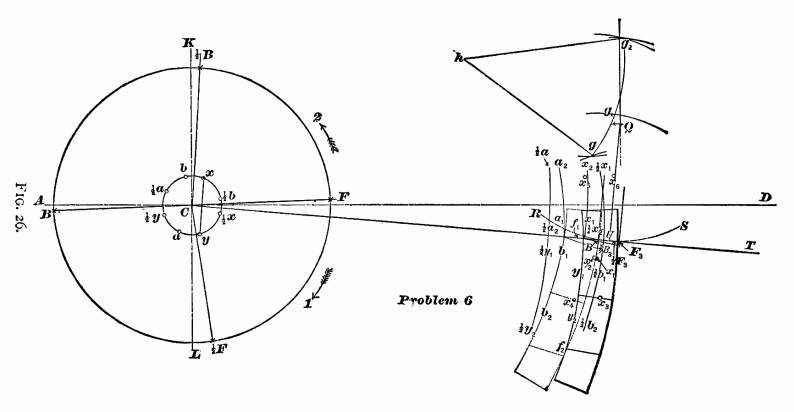For this problem we have to combine all the foregoing problems. Through the center C of axle draw the horizontal line AD, and the line KL perpendicular to it. Find the positions of crank at full and half stroke, as per Problem 1. Locate the rocker, draw the center line of motion CT, and amount of offset in lower rocker-arm, according to Problem 2. Next, locate the relative positions of eccentrics when the crank stands at full and half stroke, as explained in Problem 3. Then with a radius equal to the correct length of eccentric-rods, as explained in Problem 4, draw From the point x as a center, the arc x1 x2 From the point y as a center, the arc y1 y2 From the point ½x as a center, the arc ½x1 ½x2 From the point ½y as a center, the arc ½y1 ½y2 From the point a as a center, the arc a1 a2 From the point b as a center, the arc b1 b2 From the point ½a as a center, the arc ½a1 ½a2 From the point ½b as a center, the arc ½b1 ½b2 Locate the points ½B3 and ½F3, indicating the position of the center of lower rocker-pin when steam is cut off at half stroke; find the points x1 x2, indicating the positions of the point of suspension when the link is lifted into the position to cut off at half stroke, as explained in Problem 5, and shown in Fig. 25. Now, in order to find the position of lifting-shaft and length of arms, we must find four more additional points, —first, the position of the point of suspension of the link when the piston is at full stroke forward end of cylinder, and the crank-pin at F, the valve having z of an inch lead, and the engine moving forward, as indicated by the arrow-point I; also the position of the point of suspension of the link when the piston is at full stroke at the opposite end of the cylinder, valve z inch lead, and engine going in the same direction. To find these two points, we must know the corresponding position of the center of lower rocker-pin. In Fig. 25b 8a we see, that when the piston is at full stroke forward, and valve with inch lead, the center of valve is M of an inch in the rear of the center line of exhaust, and consequently the lower rocker-pin will be M of an inch in front of the line QU. In the same manner we can show that the center of lower rocker-pin will be M of an inch in the rear of the line QU when the piston is at the opposite end of the cylinder. Let us now locate the positions of the lower rocker-pin in Fig. 26, by drawing a line parallel to and in front of QU, with M of an inch between them: this line will intersect the arc RS in the point F3, and this point will be the center of lower rocker-pin when the piston is at full stroke forward. Draw another line M of an inch in the rear of QU and parallel to it: this line will intersect arc RS in the point B3, and this point will be the center of rocker-pin when the piston is at full stroke in the rear end of the cylinder. Now place the paper template with the line ee below the center line of motion CT, the point f1, on the arc x1 x2, the point f on the arc y1 y2, and the link-arc c3c4 just touching the point F3, and, while in this position, mark the point X of the template on the paper, which can be done with the aid of a needle, and indicate the point on the paper by x3. This point will be the position of the center of saddle-pin when the piston is at full stroke in the forward end of the cylinder, the valve having z inch lead. Again, slide the template along until the point f is on the arc a1 a2, the point f2 on the arc b1 b2, and the link-arc c3 c4 in contact with the point B3; mark the point X of the template on the paper, and indicate this point by x4. This point will be the position of the center of saddle-pin when the piston is at full stroke in the rear end of the cylinder, the valve having z inch lead. Secondly, to find the position of the point of suspension of the link when the piston is at full stroke in the forward end of the cylinder, valve having z of an inch lead, and the engine moving backward, as indicated by the arrow-point 2; also the position of the point of suspension of the link when the piston is at full stroke at the opposite end of the cylinder, valve z of an inch lead, engine going in the same direction. For this purpose, slide the template along until the line cc is above the line CT, and f in the arc a1 a2, the point f2 in the arc b1 b2 and the link-arc c3 c4 in contact with the point B3; mark the point X on the paper, and indicate this point by x5. This point will be the position of the center of saddle-pin when the piston is at full stroke in the rear end of the cylinder, valve having z of an inch lead. Again, slide the template along until the point f will be in the arc x1 x2, point f2 in the arc y1 y2, and the link-arc c3 c4 in contact with the point F3; mark the point X on the paper, and indicate this point by x6. This point will be the position of the center of saddle-pin (or the point of suspension) when the piston is at full stroke in the forward end of the cylinder, valve z of an inch lead, engine moving backward. Now, once more, with the point x3 as a center, and with the length of the link-hanger as a radius, describe an arc ; and with the point x4 as a center, and the same radius, describe another arc. These two arcs will intersect each other in the point g. Again, with the length of the link-hanger as a radius, and the points x1 x2 as centers, describe two arcs intersecting each other in the point g1, with the points x5 x6 as centers; and, with the same radius, describe another two arcs intersecting each other in the point g1. Lastly, through the points g g1 g2, draw an arc. The center h, from which the arc has been described, will be the center of the lifting-shaft, and the radius hg or hg2 will be the length of the lifting-shaft arms ; that is, the length of the two arms to which the link-hangers arc attached : the length of the other lifting-shaft arm, to which the reach-rod is attached, is made to suit the other details of the engine. When the admittance of steam ceases at the same time that the piston has reached the half stroke, the practical man would say "that the valve is cutting off equal at half stroke." When the greatest equal volume of steam is admitted alternately in each end of the cylinder, the valve is said to be cutting off equal when the link is in full gear. It is always conceded among engineers, that when the link-motion is adjusted to cut off equal at half stroke, and also to cut off equal when the link is in full gear, equal volumes of steam will be admitted alternately when the link hangs at any intermediate point. If, now, we examine Problem 5, we find, that, to obtain an equal cut-off at half stroke, it is necessary to find the proper position of saddle-pin. Again, if we examine Problem 6, we find, that, in order to obtain an equal cut-off when the link is in full gear, also an equal cut-off for any point between full gear and half stroke, we have to determine the proper position of the center of lifting-shaft and the correct length of its arms. Lastly, if we examine the first four problems, we find them simply to be preparatory problems. According to promise, we will draw attention to those points which have been, and others which have not been, approximately found. Problems 1, 2, and 3 are theoretically correct. In Problems 4, 5, and 6, the use of the template will not be admitted for theoretical reasoning; but, if the construction is made with absolute accuracy, the result will be theoretically correct. The following are a few dimensions of locomotives made by well-known makers:—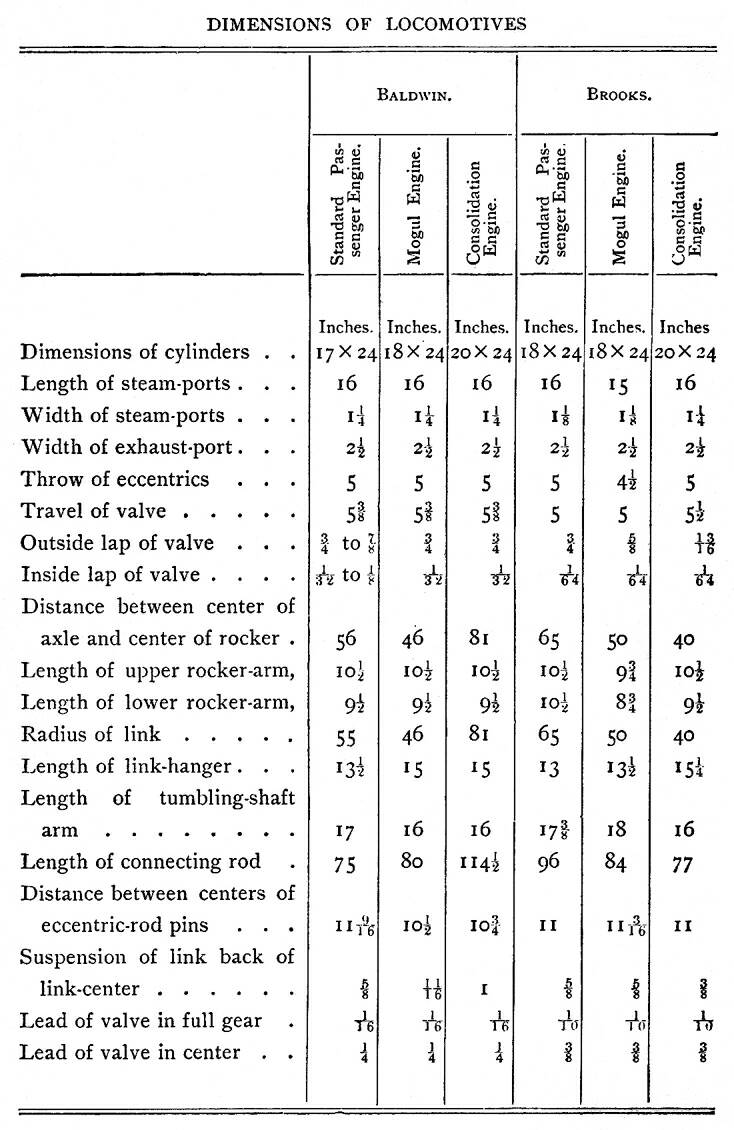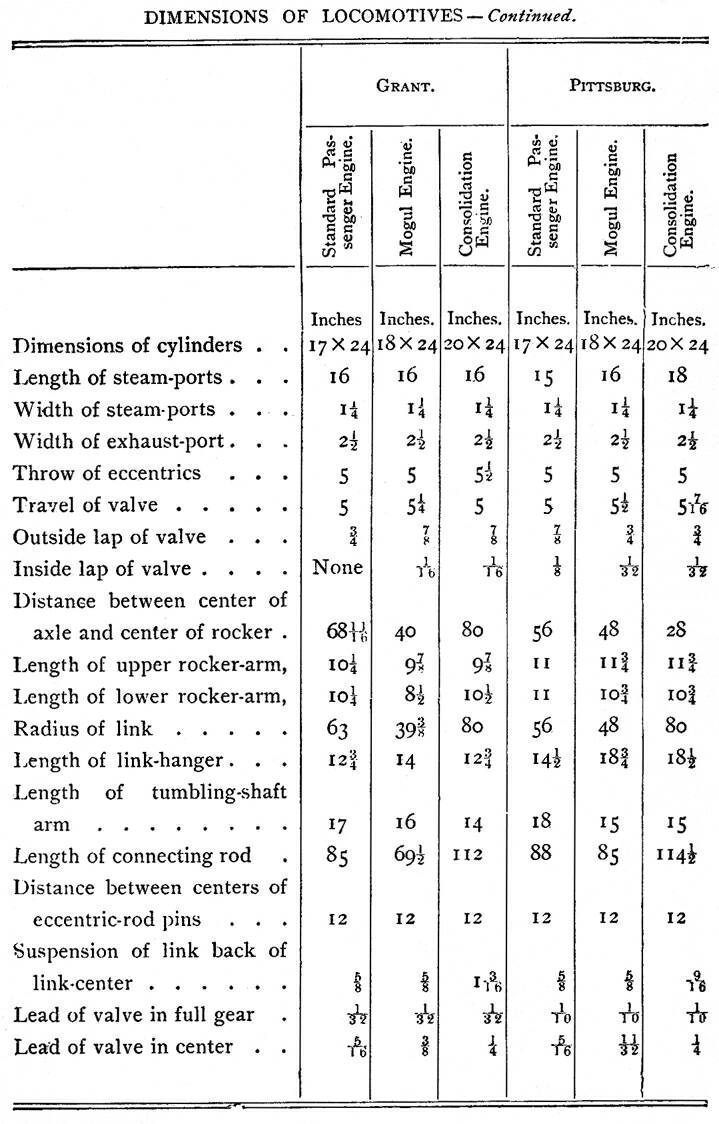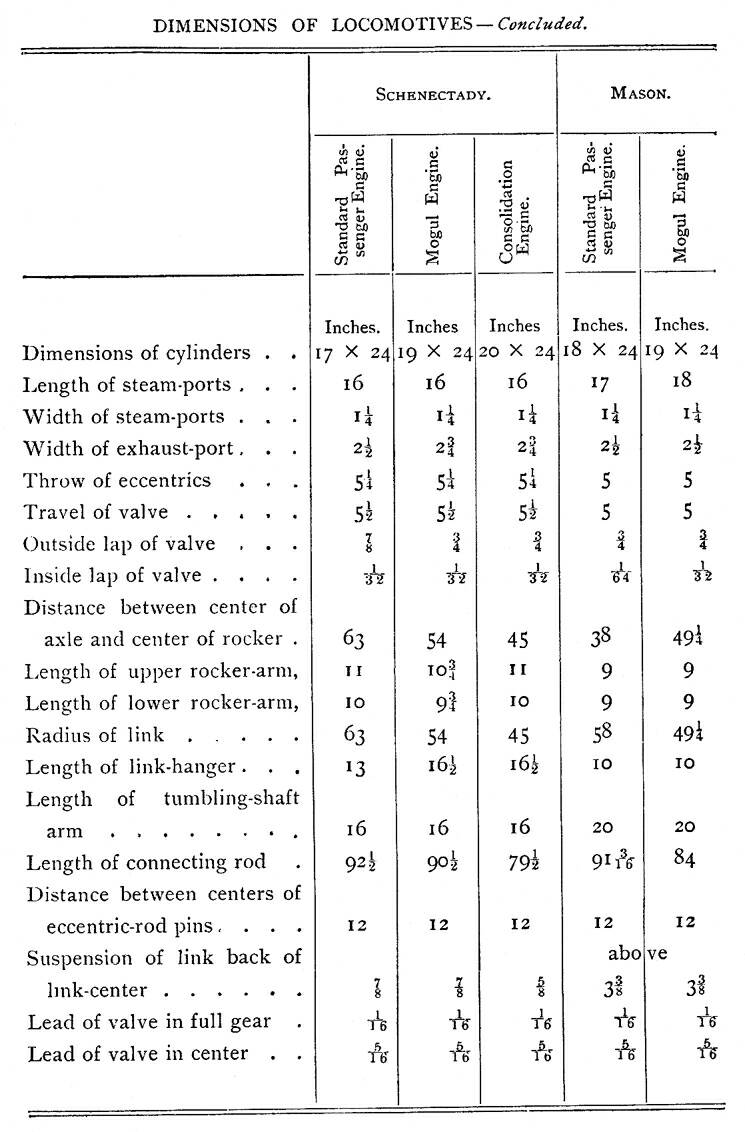Table of Contents | Contents Page